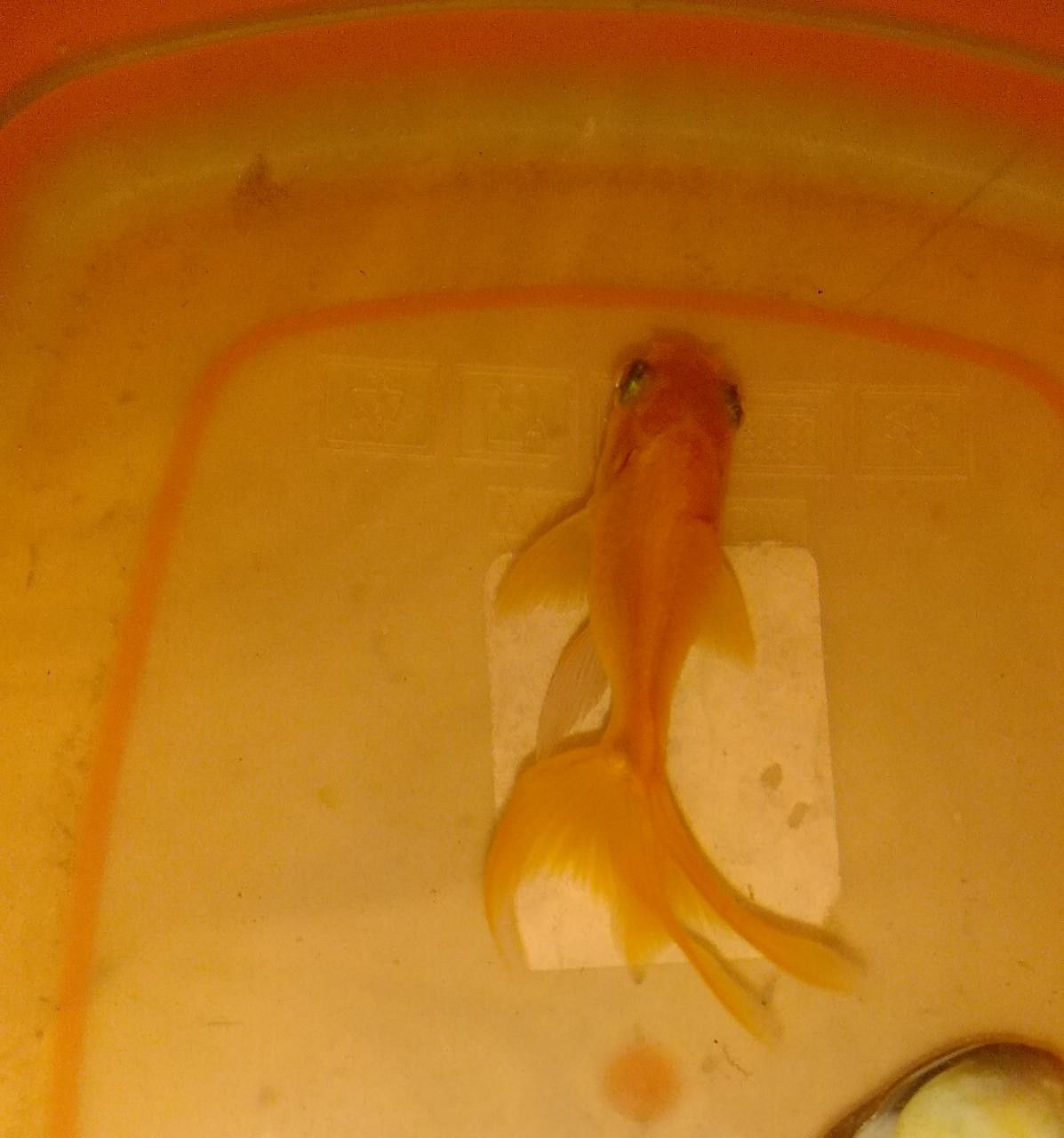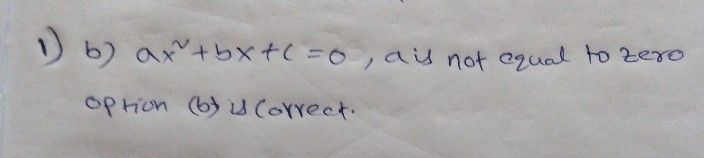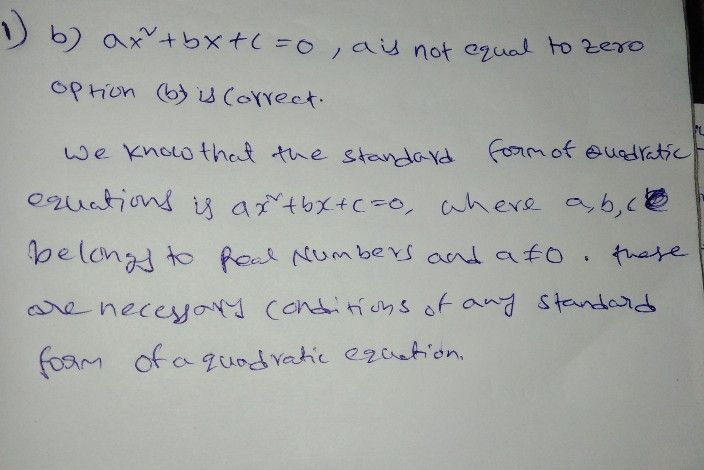Symbol
Problem$1.\right)$ Which of the following is the standard form of $48dr$ $c$ equation? $\left(I$ (Two points) $a$ $Ax+8y+c=0$ $5$ $ax^{2}+bx+c=0,als$ not equal to 0 C. $ax^{2}+bx+c<0,a$ is not equal to 0 $d$ $y=mx+b$
7th-9th grade
Other
Search count: 106
SolutionQanda teacher - NayanaQand